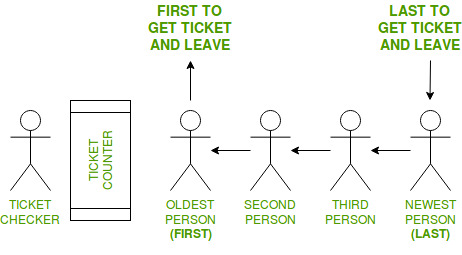# FIFO (First-In-First-Out) approach in Programming

• Difficulty Level : Basic
• Last Updated : 20 Jul, 2021

FIFO is an abbreviation for first in, first out. It is a method for handling data structures where the first element is processed first and the newest element is processed last.
Real life example:Attention reader! Don’t stop learning now. Get hold of all the important DSA concepts with the DSA Self Paced Course at a student-friendly price and become industry ready.  To complete your preparation from learning a language to DS Algo and many more,  please refer Complete Interview Preparation Course.

In case you wish to attend live classes with experts, please refer DSA Live Classes for Working Professionals and Competitive Programming Live for Students.

In this example, following things are to be considered:

• There is a ticket counter where people come, take tickets and go.
• People enter a line (queue) to get to the Ticket Counter in an organized manner.
• The person to enter the queue first, will get the ticket first and leave the queue.
• The person entering the queue next will get the ticket after the person in front of him
• In this way, the person entering the queue last will the tickets last
• Therefore, the First person to enter the queue gets the ticket first and the Last person to enter the queue gets the ticket last.

This is known as First-In-First-Out approach or FIFO.
Where is FIFO used:

1. Data Structures
Certain data structures like Queue and other variants of Queue uses FIFO approach for processing data.

2. Disk scheduling
Disk controllers can use the FIFO as a disk scheduling algorithm to determine the order in which to service disk I/O requests.

3. Communications and networking
Communication network bridges, switches and routers used in computer networks use FIFOs to hold data packets en route to their next destination.

Program Examples for FIFO
Program 1: Queue

## C++

 `// C++ program to demonstrate``// working of FIFO``// using Queue interface in C++` `#include``using` `namespace` `std;` `// print the elements of queue``void` `print_queue(queue<``int``> q)``{``    ``while` `(!q.empty())``    ``{``        ``cout << q.front() << ``" "``;``        ``q.pop();``    ``}``    ``cout << endl;``}` `// Driver code``int` `main()``{``    ``queue<``int``> q ;` `    ``// Adds elements {0, 1, 2, 3, 4} to queue``    ``for` `(``int` `i = 0; i < 5; i++)``        ``q.push(i);` `    ``// Display contents of the queue.``    ``cout << ``"Elements of queue-"``;``        ` `    ``print_queue(q);` `    ``// To remove the head of queue.``    ``// In this the oldest element '0' will be removed``    ``int` `removedele = q.front();``    ``q.pop();``    ``cout << ``"removed element-"` `<< removedele << endl;` `    ``print_queue(q);` `    ``// To view the head of queue``    ``int` `head = q.front();``    ``cout << ``"head of queue-"` `<< head << endl;` `    ``// Rest all methods of collection interface,``    ``// Like size and contains can be used with this``    ``// implementation.``    ``int` `size = q.size();``    ``cout << ``"Size of queue-"` `<< size;``        ` `    ``return` `0;``}` `// This code is contributed by Arnab Kundu`

## Java

 `// Java program to demonstrate``// working of FIFO``// using Queue interface in Java` `import` `java.util.LinkedList;``import` `java.util.Queue;` `public` `class` `QueueExample {``    ``public` `static` `void` `main(String[] args)``    ``{``        ``Queue q = ``new` `LinkedList<>();` `        ``// Adds elements {0, 1, 2, 3, 4} to queue``        ``for` `(``int` `i = ``0``; i < ``5``; i++)``            ``q.add(i);` `        ``// Display contents of the queue.``        ``System.out.println(``"Elements of queue-"` `+ q);` `        ``// To remove the head of queue.``        ``// In this the oldest element '0' will be removed``        ``int` `removedele = q.remove();``        ``System.out.println(``"removed element-"` `+ removedele);` `        ``System.out.println(q);` `        ``// To view the head of queue``        ``int` `head = q.peek();``        ``System.out.println(``"head of queue-"` `+ head);` `        ``// Rest all methods of collection interface,``        ``// Like size and contains can be used with this``        ``// implementation.``        ``int` `size = q.size();``        ``System.out.println(``"Size of queue-"` `+ size);``    ``}``}`

## Python3

 `# Python program to demonstrate``# working of FIFO``# using Queue interface in Java` `q ``=` `[]` `# Adds elements {0, 1, 2, 3, 4} to queue``for` `i ``in` `range``(``5``):``    ``q.append(i)` `# Display contents of the queue.``print``(``"Elements of queue-"` `, q)` `# To remove the head of queue.``# In this the oldest element '0' will be removed``removedele ``=` `q.pop(``0``)``print``(``"removed element-"` `, removedele)` `print``(q)` `# To view the head of queue``head ``=` `q[``0``]``print``(``"head of queue-"` `, head)` `# Rest all methods of collection interface,``# Like size and contains can be used with this``# implementation.``size ``=` `len``(q)``print``(``"Size of queue-"` `, size)` `# This code is contributed by patel2127.`

## C#

 `// C# program to demonstrate``// working of FIFO``using` `System;``using` `System.Collections.Generic;` `public` `class` `QueueExample``{``    ``public` `static` `void` `Main(String[] args)``    ``{``        ``Queue<``int``> q = ``new` `Queue<``int``>();` `        ``// Adds elements {0, 1, 2, 3, 4} to queue``        ``for` `(``int` `i = 0; i < 5; i++)``            ``q.Enqueue(i);` `        ``// Display contents of the queue.``        ``Console.Write(``"Elements of queue-"``);``        ``foreach``(``int` `s ``in` `q)``                ``Console.Write(s + ``" "``);` `        ``// To remove the head of queue.``        ``// In this the oldest element '0' will be removed``        ``int` `removedele = q.Dequeue();``        ``Console.Write(``"\nremoved element-"` `+ removedele + ``"\n"``);``        ``foreach``(``int` `s ``in` `q)``                ``Console.Write(s + ``" "``);` `        ``// To view the head of queue``        ``int` `head = q.Peek();``        ``Console.Write(``"\nhead of queue-"` `+ head);` `        ``// Rest all methods of collection interface,``        ``// Like size and contains can be used with this``        ``// implementation.``        ``int` `size = q.Count;``        ``Console.WriteLine(``"\nSize of queue-"` `+ size);``    ``}``}` `// This code has been contributed by 29AjayKumar`

## Javascript

 ``

Output:

```Elements of queue-[0, 1, 2, 3, 4]
removed element-0
[1, 2, 3, 4]